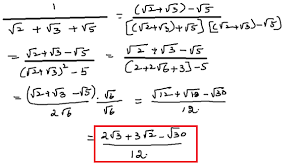Wellcome!

Question 2. Asked on :12 January 2019:05:55:36 PM

add √125 + 2√27 and -5√5 - √3

-Added by khushi chauhan Mathematics » Number System

Akki chauhan

√125 +2√27 +(-5√5 -√3)

=√5×5×5 +2√3×3×3 -5√5 -√3

=5√5 +6√3 -5√5 -√3

=5√3

-Answered by Akki chauhan On 15 January 2019:09:05:29 AM

Question 3. Asked on :12 January 2019:05:54:25 PM

Rationalise the denominator = 1√3+√5+√7

-Added by Himanshi Verma Mathematics » Number System

priyanshu kumar-Answered by priyanshu kumar On 17 February 2020:02:57:05 PM

Question 4. Asked on :12 January 2019:10:52:27 AM

Find the zero of 2x-5.

-Added by Akki chauhan Mathematics » Number System

Master Purushottam

2x-5=0

2x=5

x= 5/2

-Answered by Master Purushottam On 22 August 2019:09:11:57 PM

Question 5. Asked on :09 January 2019:09:37:10 AM

Ques.1. prove that 2+2= 5

-Added by Master Purushottam Mathematics » Number System

Nishant Verma

Which is the same as: 16-36 = 25-45
Which can also be expressed as: (2+2) 2 (9 X (2+2) = 52) 9 X 5
Add 81/4 to both sides: (2+2) 2 (9 X (2+2) + 81/4 = 52) 9 X 5 + 81/4
Rearrange the terms: ({2+2}) 9/2) 2 = (5-9/2) 2
Ergo: 2+2 - 9/2 = 5
Hence: 2 + 2 = 5

-Answered by Nishant Verma On 13 October 2019:09:38:52 AM

Question 6. Asked on :09 January 2019:09:36:20 AM

What is Euclid's division algorithm.

-Added by Master Purushottam Mathematics » Number System

Nishant Verma
The basis of the Euclidean division algorithm isEuclid's division lemma. To calculate the Highest Common Factor (HCF) of two positive integers a and b we use Euclid's division algorithm. HCF is the largest number which exactly divides two or more positive integers.

-Answered by Nishant Verma On 17 October 2019:02:37:10 PM

You can see here all the solutions of this question by various user for NCERT Solutions. We hope this try will help you in your study and performance.

This Solution may be usefull for your practice and CBSE Exams or All label exams of secondory examination. These solutions or answers are user based solution which may be or not may be by expert but you have to use this at your own understanding of your syllabus.

What do you have in your Mind....

* Now You can earn points on every asked question and Answer by you. This points make you a valuable user on this forum. This facility is only available for registered user and educators.

Do you have a question to ask?

User Earned Point: Select

All Tags by Subjects:

Science (2235)
History (278)
Geography (310)
Economics (258)
Political Science (96)
Mathematics (201)
General Knowledge (5686)
Biology (94)
Physical Education (20)
Chemistry (118)
Civics (114)
Home Science (12)
Sociology (9)
Hindi (45)
English (259)
Physics (1435)
Other (97)
Accountancy (378)